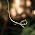2011年8月7日 星期日

Python 的特別之處 (1)

list、dictionary and string

In : for ch in "abcd":
....:         print ch
....:
a
b
c
d

iterator

In : for i, n in enumerate([1, 3, 5]):
....:     print i, n
....:
0 1
1 3
2 5

In : names = ["John", "Marry", "Tom"]
In : sexes = ["Male", "Female", "Male"]
In : for name, sex in zip(names, sexes):
....:     print name, sex
....:
John Male
Marry Female
Tom Male

map, filter and reduce

In : map(int, ["12", "37", "999"])
Out: [12, 37, 999]
In : map(str, [12, 37, 999])
Out: ['12', '37', '999']

int 是一個函式，將傳入的物件轉成整數；str 則是轉成字串。使用 map 可以將一個 iterator 轉為另一種 list。

In : numbers = [1, 2, 3, 4, 5]
In : filter(lambda x: x % 2 == 0, numbers)
Out: [2, 4]

In : numbers = [1, 2, 3, 4, 5]
In : reduce(lambda x, y: x + y, numbers, 0)
Out: 15

In : bits = [0, 1, 0, 0, 1]  # bits[i] 的值表示 2^i 的系數

In : reduce(lambda x, (i, b): x | (b << i), enumerate(bits), 0)
Out: 18

list comprehension

map 和 filter 雖然方便，要用到 lambda 或是混合使用時就沒那麼好讀了。Python 提供一個重量級的武器 list comprehension 來解決這問題。比方說留下偶數並乘以三再加一：

In : numbers = [1, 2, 3, 4, 5]

In : [n * 3 + 1 for n in numbers if n % 2 == 0]
Out: [7, 13]

def qsort(numbers):
if len(numbers) <= 1:
return numbers
pivot = numbers
rest = numbers[1:]
smaller = [n for n in rest if n <= pivot]
larger = [n for n in rest if n > pivot]
return qsort(smaller) + [pivot] + qsort(larger)

tuple

tuple 是一個很妙的資料結構，它和 list 的主要差別是它是唯讀的，Python 裡鮮少有這種唯讀物件。不過它較易發覺的好處是被用在 Python 的 parallel assignment 和函式傳回值。

a, b = b, a # swap

Python 在看到 b, a 時會產生一個 tuple 表示 (b, a)，再透過 tuple 達到 parallel assignment

In : def divide_and_mode(a, b):
...:     if b == 0:
...:         return None, None
...:     return a / b, a % b
...:

In : divide_and_mode(7, 3)
Out: (2, 1)

In : a, b = divide_and_mode(7, 3)

In : a
Out: 2

In : b
Out: 1

with

# 印出所有使用者的 id
with open('/etc/passwd') as fr:
for line in fr:
print line.split(':')

簡單不易出錯的語法

if (condition);
{
// BUG!! 這裡的程式一定會被執行
}
if (x < 60)
number_of_fail++;
total_fail_score += x; // BUG!! 這行每次都會執行

3 則留言:

1.這些在c#, vb, ruby 都有, 老實說也沒啥特別的.

1.針對樓上的回覆，我想...很多語言都有其相似之處吧~
身為一個python初學者，我覺得這篇文章相當受用! 謝謝你:)

2.簡明又實用的平行處理技巧, 讚!